# Switching power supply conduction disturbance, solution to conducted disturbance

Share
/ 2019-03-21 / Tech

1 Overview

At present, the electromagnetic compatibility of electronic products is receiving more and more attention, especially in developed countries. A complete electromagnetic compatibility system has been formed, and China is also establishing an electromagnetic compatibility system. Therefore, achieving electromagnetic compatibility of products is a passport to enter the international market. For the switching power supply, since the switching tube and the rectifier tube operate under large current conditions and high voltage conditions, strong electromagnetic interference is generated to the outside world, so the switching power supply Conducted emissions and electromagnetic radiation emissions are more difficult to achieve electromagnetic compatibility than other products, but if we understand the principle of electromagnetic interference from switching power supplies,It is not difficult to find a suitable countermeasure to reduce the conducted emission level and the radiation emission level to an appropriate level to achieve electromagnetic compatibility design.

2 Switching Power Conduction Disturbance

2.1 Conducted emission generation

Conductive disturbance of switching power supply is through power supply Enter the electromagnetic interference that the power line is propagating outward. The harassment that propagates outward in the input power line of the switching power supply has both differential mode disturbance and common mode disturbance. The common mode disturbance produces stronger radiation disturbance than the differential mode disturbance. The test frequency of conducted disturbance is in the range of 150KHz to 30MHz. The limit requirements are as shown in Table 1 below: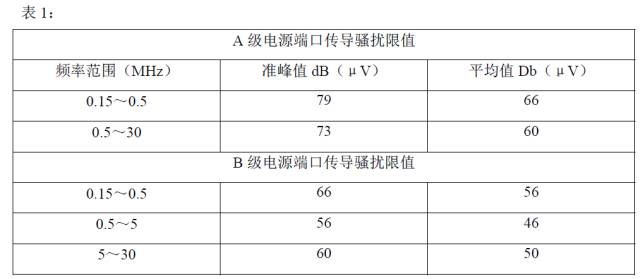In the frequency range of 0.15MHz~1MHz, the disturbance mainly exists in the form of common mode. In the frequency range of 1MHz~10MHz, the form of disturbance is the coexistence of differential mode and common mode, at 10MHz. Above, the form of harassment is mainly based on the common film. The differential mode disturbance of conducted emission is mainly caused by the switching tube operating in the switching state. When the switching tube is turned on, the current flowing through the power line rises linearly, and the current suddenly changes to 0 when the switching tube is turned off, so the current flowing through the power line The high-frequency triangular pulsating current is rich in high-frequency harmonic components. As the frequency increases, the amplitude of the harmonic component becomes smaller and smaller, so the differential mode disturbance decreases with the increase of the frequency. As shown in Figure 1, due to the presence of capacitor C5,It forms a low-pass filter with the inductorL3. Therefore, the differential mode conduction disturbance mainly exists in the low frequency range.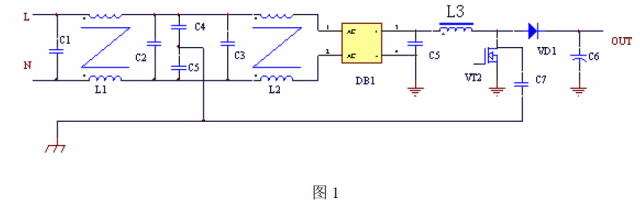The main cause of common mode disturbance is that there is a distributed capacitance between the power supply and the earth (protective ground), and the high-frequency harmonic component of the square wave voltage passes through the circuit The distributed capacitance is transmitted to the earth and forms a loop with the power line, causing common mode disturbance.

As shown in Figure 1, L, N are the power input, C1, C2, C3, C4, C5, L1, L2 form the input EMI filter, DB1 For the rectifier bridge, L1, VD1, C6 and VT2 are power factor correction main circuits,VT2 is a switch tube, and the D pole of the switch tube is connected to the heat sink of the tube. When the switch tube is mounted on the heat sink, a capacitance is formed between the heat sink and the heat sink, as shown by C7 in FIG. It is shown that the switching tube VT2 operates in the switching state, the voltage of the D pole is a high frequency square wave, the frequency of the square wave is the switching frequency of the switching tube, and the harmonics of the square wave pass through the coupling capacitor, the L, N power source. The lines form a loop that creates common mode disturbances. The distributed capacitance of the power supply and the earth is relatively scattered and difficult to estimate, but from the above Figure 1, the coupling capacitor between the D pole of the switching tube VT2 and the heat sink has the greatest effect. In Figure 1 above, from the rectifier bridge to the inductor The voltage between L3 is a 100Hz power frequency waveform, and the voltage between the inductor L3 and the diode VD1 and the VT2D pole of the switch is a square wave voltage, which contains a large number of high harmonics. wave. Secondly, the influence of the inductor L3 is relatively large, but the distance between the L3 and the casing is far, and the distributed capacitance is much smaller than the coupling capacitance between the switch tube and the heat sink. Therefore, we mainly consider the coupling capacitance between the switch tube and the heat sink. .

2.2 Solutions to Conducted Harassment

2.2.1 EMI Filter

Resolving conducted disturbances Mostly, passive filters are used. As shown in Figure 1, C1, C2, C3, C4, C5, L1, and L2 form an EMI filter. L1 and L2 are two common-mode inductors. Generally, in the common-mode inductor, there are about 20% of differential-mode inductors, and capacitors C1, C2, and C3 form a differential-mode filter, and C4 and C5 are common-mode capacitors. A common mode filter is formed with the inductors L1 and L2.

Computation of common mode inductance:

Assume that the interference voltage of the collector of the switch is About 400V, converted to dB (μV) is: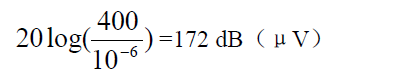The internal decoupling network (LISN) internal conduction resistance of the conducted emission test equipment is 50Ω. Then the coupling capacitor C7 and the internal resistance Zin of the test equipment decoupling network attenuate the disturbance level:

Yes: If the EMI filter is not added, the disturbance output measured by the power output port Ping is: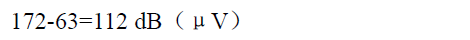The Class A power port conduction limit requirement in Table 1 is 79 dB(μV), which clearly exceeds the limit requirements. The attenuation of the filter at 150KHz is required:

112-79=33 dB, considering at least 6dB margin, the EMI filter is at 150KHz The attenuation should be greater than 39dB, we take 40dB. The attenuation characteristic of the second-order filter is -40dB/10 octave. In Figure 1, there are two second-order filters with attenuation characteristics of -80dB/10 octave. The corner frequency of the filter should be around: 47KHz. To the influence of other factors,The corner frequency of the filter is taken as 40 kHz.

Common mode capacitor C4, C5 take 4700P (taking into account the leakage current problem, can not take too much), then: C = C4 + C5 = 9400P.

Calculated: L=1.7mH

When designing an EMI filter, in order to effectively suppress the disturbance signal, the source impedance to be connected at both ends of the filter must be properly matched. When the output impedance Zo and the load impedance RL of the filter are not equal,Generated at this port, the reflection coefficient ρ is defined by:

The greater the difference between Zo and RL, the greater the reflection from the port.

The common mode inductor in the EMI filter contains about 20% differential mode inductance, and the X2 capacitor forms a differential mode filter. In the above schematic, The X2 capacitors C1, C2, and C3 have a large influence on the low-frequency side of the conducted disturbance. The main reason is that in the low frequency band, the mode of disturbance mainly exists in the differential mode. Increasing C1, C2, and C3 can reduce the low frequency band. The level of disturbance, but the value generally does not exceed 0.47 ~ 2.2μF, if the capacitor is properly increased, the low frequency band is still exceeding the standard, and the differential mode inductance can be added to solve.

2.2.2 Other methods

EMI filter is a method of cutting off the propagation path Reduce the level of disturbance of conducted emissions, and we can also start from the source of the emission to reduce the level of emission from the source.

1: As shown in Figure 2 below:

In Figure 2, the PFC boost inductor Adding an auxiliary winding, the winding has the same number of turns as the main winding, the direction is opposite to the main winding, and C7 is the coupling capacitance between the switching tube and the heat sink.Add a capacitor similar to the C7 capacity to the heat sink and the auxiliary winding as shown in the figure, so that the amplitude of the disturbance signal coupled to the heat sink by C7 and C8 is the same, and the two signals just cancel each other out. Reduce the level of disturbance emitted by the outward emission.

2: as shown in Figure 3 below:

In Figure 3, add a high-frequency capacitor C8, connected to the switch Between the heat sink and the output ground, the connection between the capacitor and the heat sink is as close as possible to the switch tube. The capacitor is a safety capacitor with a capacity of 4700P to 0.01μf. If the capacitance is too large, the leakage current of the power supply will exceed the standard. The disturbance signal coupled to the heat sink by capacitor C7 is attenuated by C8.The coefficient of attenuation is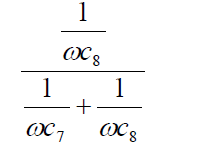Because C8 is much larger than C7, the above formula can be simplified as:Visible, assuming C7 is 30P, C8 For the 4700P, the outburst signal is attenuated by 157 times, nearly 45dB.

3 Radiation Disturbance of Switching Power Supply

3.1 Space Transmission of Radiation Disturbance

1. Far and Near Field

Electromagnetic energy propagates around the field in the form of a field, which forms radiated disturbances. The field can be divided into near-field and far-field, and near-field is also called induction. Field, its nature is closely related to the field source. If the field source is a source of high voltage and small current, the near field is mainly an electric field. If the field source is a low voltage and a large current, the field source is mainly a magnetic field. Whether the near field is a magnetic field or an electric field, when the distance from the field source is greater than λ/2π, it becomes a far field, also known as a radiation field.

Because the switching power supply operates at high voltage and high current, there is an electric field and a magnetic field in the near field.

2. Radiation methods of harassment

● Single-point radiation, mainly simulating the sameness The smaller source of radiation, the intensity of the radiation can be expressed as:

where P is the transmitted power and r is the distance from the source. It can be seen that the single point radiation intensity is inversely proportional to the distance and proportional to the square root of the power of the source.

● Radiation of a parallel two-wire loop

Mainly simulates the radiation of a differential mode current loop Source, its radiation intensity can be expressed as:

where A is the area enclosed by the differential mode current, I is the magnitude of the differential mode current, and r is the distance from the source. λ is the wavelength. It can be seen that the differential mode radiation intensity is proportional to the magnitude of the differential mode current and the area surrounded by the differential mode current, inversely proportional to the distance, and proportional to the square of the frequency.

Therefore, high-frequency decoupling capacitors should be added to the high-frequency noise source to prevent high-frequency noise from flowing into the power supply loop.

The single-wire radiation formula can be used to estimate common mode The amount of radiation generated by the current: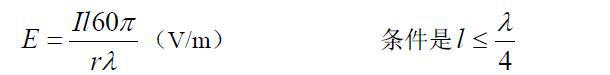where I is the magnitude of the common mode current, r is the distance to the common mode current source, l is the length of the wire, and λ is the wavelength.

3. Common mode current radiation

Two similar wires, if they flow poorly Mode current, the electromagnetic field generated by the wire is reversed,Equal in size and cancel each other out, but if a common mode current flows, the electromagnetic fields generated by the two wires overlap each other. Therefore, the spatial radiation generated by the common mode current of the same size is much larger than the spatial radiation intensity generated by the differential mode current. According to the experiment, the radiation intensity of the two is different by a thousand times. Therefore, the radiation of the switching power supply is mainly caused by the common mode current.

● Basic mode of common mode current radiation

Common mode radiation has two drive modes One is the current drive mode, and the other is the voltage drive mode. In the switching power supply, the main function is the voltage drive mode.

● Conditions for generating common mode radiation

There are two conditions for generating common mode radiation. One is a common mode drive source and the other is a common mode antenna.

There is a radio frequency potential difference between any two metal bodies, which constitutes an asymmetric vibrator antenna. The two metal conductors are divided into two parts.

Do not be two antennas Extremely, for a switching power supply, as shown below:

C7 in Figure 4 is the coupling capacitance between the switch tube and the heat sink. The heat sink is connected to the D pole of the switch tube. The trace of the antenna is the two poles of the antenna, which can be simplified to Figure 5 below:In the figure, Vs is the source of disturbance, and for Figure 4, it is the switch The D pole of the tube VT2, L1, L2 is equivalent to the two poles of the antenna, one pole is the printed line connected to the D pole of the switch tube, the other pole is the heat sink and the grounding wire connected thereto, and C is the pole of the antenna The coupling capacitance between the switch and the heat sink in Figure 4.

Common mode radiation mainly has the magnitude of the common mode current on the antenna. Therefore, the larger the coupling capacitance between the two poles L1 and L2, the greater the radiated power.

In addition, when the total length of the two poles of the antenna When it is larger than λ/20, energy can be radiated outward, and the radiation energy is maximized when the length of the antenna and the wavelength of the disturbance source satisfy the following conditions.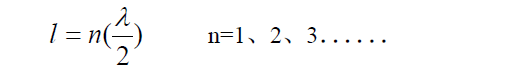3.2 Radiation source of switching power supply

To solve and reduce the electromagnetic radiation of switching power supply, first understand the radiation of switching power supply The source is there. For a switching power supply with a PFC power factor correction circuit in the front stage, the source of the radiated disturbance is mainly distributed in the following places (radiation sources such as drivers in the switching power supply are much weaker than those listed below, so Can not be considered).

1. PFC switch tube

2.PFC boost diode

3. DC/DC switch tube

4. DC/DC rectifier, freewheeling tube

5. PFC boost inductor

6. DC/DC Transformer

● The radiation principle of PFC switch and DC/DC switch is as above As mentioned, the drive source belonging to the voltage drive mode, the boost inductor and the transformer belong to the differential mode disturbance source. The main reason is the presence of leakage inductance, which causes electromagnetic energy to leak and emit electromagnetic energy outward.

● When the PFC boost diode and DC/DC rectifier diode are turned off,There is a reverse recovery current, as shown below:

The figure shows the reverse recovery current of the actual tested PFC boost diode turn-off instant (without absorption) In Figure 4, the reverse recovery current mainly forms a loop through C6, VD1, and VT2 to form differential mode radiation. In addition, due to the presence of lead inductance, a small portion of the current passes between the heat sink and the switching transistor VT2. The coupling capacitor C7 flows outward to form common mode radiation.

The reverse recovery current of the rectifier diode and freewheeling tube of DC/DC will cause a high voltage spike in the reverse voltage of the diode. Figure 7 below is positive. The output filter circuit of the circuit.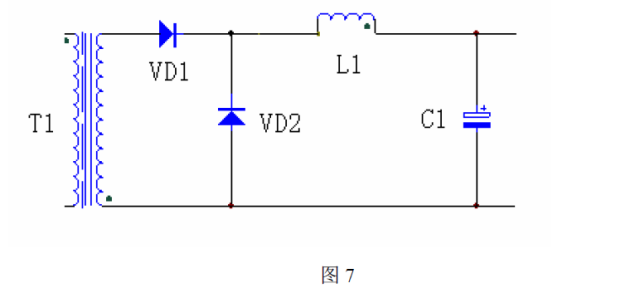In Figure 7, TI is the transformer, and VD1 and VD2 are the rectifier and freewheel respectively, because the rectifier and freewheel are turned off by conduction. There is a reverse recovery current, which generates a relatively high voltage peak across VD1 and VD2. Since the reverse recovery current of the fast recovery diode is in the range of several tens of nS, the peak voltage has a higher frequency and its fundamental frequency. At tens of MHz, due to the high frequency, the radiation capability is very strong. Figure 8 below shows the voltage waveform of the rectifier and freewheel.In Figure 7, the rectifier and freewheel are fixed to the radiator The heat sink is connected to the earth. Since the cathode of the diode is directly connected to the heat sink of the tube shell, a coupling capacitor is formed between the heat sink of the tube shell and the heat sink, and the high voltage peak generated by the rectifier tube and the freewheel tube at the time of the cutoff is Common mode radiation is generated by the coupling capacitor flow, and the output line and ground are the two poles of the antenna respectively.

●Switching power supply Other radiation sources such as printed lines The common mode radiation caused by the distributed capacitance between the chassis and the differential mode radiation generated by the internal circuit operation is much smaller than the previous several radiation sources.

3.3 Solutions to Radiation Harassment

The above analysis of the causes of radiation disturbance and the radiation source of the switching power supply, it is easier to solve the radiation problem of the switching power supply.

3.3.1 Switch Radiation emission caused by tube emission source

The conduction disturbance of the input port described above is emitted outward through the input line, and the input line is another Antenna, when the common mode current flows through the input line, it will emit electromagnetic energy to the space and generate radiated disturbance. Therefore, for the above measures to solve the conducted emission, the conductive emission is reduced and the input port is greatly reduced. Radiation emission.

For the radiation source DC/DC switch, the same measures as the PFC switch can be used to reduce the voltage amplitude of the drive source. , the intensity of smaller radiation emissions.

Figure 9 below is a comparison of the radiant intensity of the PFC switch tube heat sink to the PFC output ground.

In the picture, the front is the capacitor, the back is Without capacitors, it can be seen from the two graphs that the radiation disturbance level is reduced by 10 DB after adding capacitance in the vicinity of 50 MHz, and is also reduced by about 10 DB in the frequency range of 120 MHz to 220 MHz.

3.3.2 DC/DC rectifier, freewheel source

for DC/DC rectifiers , continuous flow tube emission source, in addition to increasing absorption,In addition to reducing the peak voltage across the diode and saturating the magnetic ring on the diode pins to reduce the reverse recovery current, the following measures can be taken.

1. Connect the grounding capacitor to the output ground near the contact point of the rectifier, freewheel and heat sink, as shown in Figure 10 below: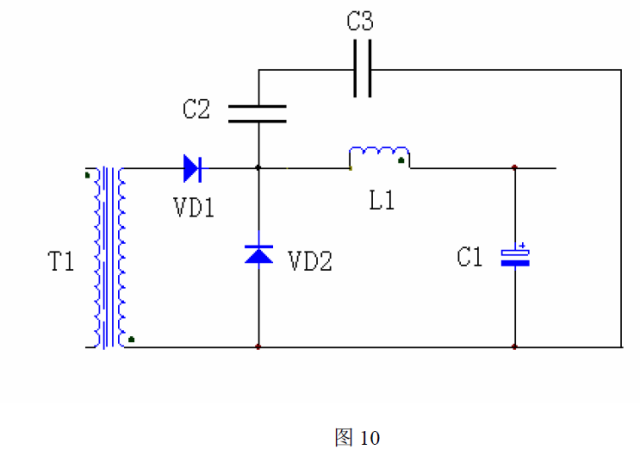C2 in the figure is the coupling capacitance between the diodes VD1 and VD2 and the heat sink. The capacity is generally tens of PF, C3 is the increased capacitance, C3 is much larger than C2, DC/DC rectifier, freewheeling tube The voltage peak is divided by C2 and C3, and the amplitude is greatly reduced, which can greatly reduce the outward radiation.

2. Use the circuit form shown in Figure 11 below.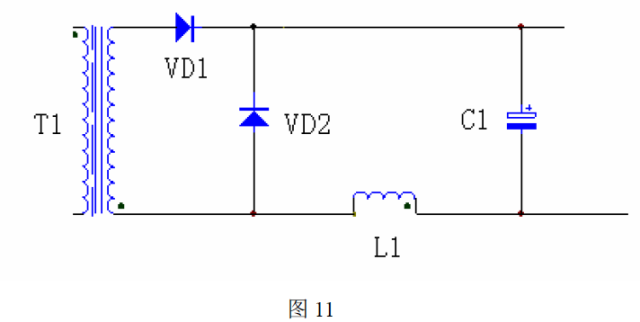In the circuit form of the above figure, the output filter inductor is placed at the negative end of the output, and the outputs of VD1 and VD2 are directly connected to the positive terminal of the output filter capacitor. Thus, the rectifier and freewheeling The cathode of the tube is connected to a fixed level, and the common mode current flowing outward through the coupling capacitance between the heat dissipating surface of the cathode and the heat sink is greatly reduced, thereby greatly reducing the radiation level of the output port.

3.3.3 chassis shielding

The radiation of the switching power supply is radiated directly from the input and output ports except the above-mentioned radiation source. The control circuit, drive, auxiliary power supply, transformer, and inductor of the power supply directly radiate electromagnetic energy to the space. Therefore, the chassis needs to be shielded. Shielding should consider the impact of the material, thickness and hole spacing of the chassis on shielding effectiveness.

1. Absorption loss

When the electromagnetic wave enters the metal shield, it will induce current and become heat energy, so the electromagnetic wave enters the metal conductor and decays exponentially, and the transmission distance is very short.

We attenuate the electromagnetic wave to the original 1/e, which is 0.37 times the distance called the skin depth δ

set The skin depth δ is related to the material's performance and frequency and can be expressed by the following formula: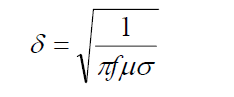In the formula, μ is the permeability of the material and σ is the conductivity of the material.

2. Reflection loss

When electromagnetic waves reach the surface of both media, the impedance is not The electromagnetic wave energy loss caused by the reflection and the reflection is called the reflection loss.

The frequency range tested by radiated disturbance is 30MHz to 1000MHz. If only electromagnetic shielding above 30MHz is considered, a thin layer of conductor can achieve high shielding effectiveness, but for a relatively low frequency electric or magnetic field,It is necessary to consider the materials and thicknesses used for shielding.

3. Effect of hole seams on shielding

In practical applications, on the chassis There are always connection holes, ventilation holes and connection gaps between the faces of the chassis. If the hole size of the chassis is unreasonable, the shielding effectiveness will be greatly reduced. Generally, the size of the hole should be less than one tenth. One percent of the wavelength can achieve the corresponding shielding effect. If the upper limit frequency is considered at 1000MHz, the size of the hole slit should be less than: 3 to 0.3cm. Since the electromagnetic radiation frequency range of the switching power supply is generally between 30MHz and 500MHz, the upper limit frequency of the shielding can be considered at 500MHz.

Relevant articles recommend

Top suppliers

Product:Photo Coupler,Optocoupler,IC,TOS,ON,AVAGO,SHARP,RENESAS,CT,EL,LTV,TI,NXP,ST
1YRS
Product:Infrared Receiver Module,Infrared Emitting Diode,Silicon PIN Photodiode,Silicon Phototransistor,LED,Silicon Photocell,Hall Effect IC,Photoelectric switch,Photoelectric encoder,IC.
1YRS
Product:KSD301 KSD9700 thermostat thermal protector fuse sensor
1YRS
Product:IC,PCB,module,capacitor,resistor,diodes,LED,LCD
1YRS
Product:Electronics components，ICs，MCU，RS-485/RS-422 Transceiver
9YRS
Subscribe News